## What is the Analytical/Window Function?

• Window functions operate on a set of rows and return a single value for each row from the underlying query.
• The term window describes the set of rows on which the function operates.
• A window function uses values from the rows in a window to calculate the returned values.
• A query can include multiple window functions with the same or different window definitions.
• There are two types of window functions: Aggregate functions and Analytical functions.
##### Over() clause
• When we use a window function in a query, define the window using the OVER() clause.
• The OVER() is a mandatory clause that defines a window within a query result set.
• The OVER() clause has the following capabilities:
• Defines window partitions to form groups of rows (PARTITION BY clause).
• Orders rows within a partition (ORDER BY clause).
• Syntax:

```window_function (expression) OVER (
[ PARTITION BY expr_list ]
[ ORDER BY order_list ][ frame_clause ] )```
##### Partition By:
• PARTITION BY is an optional clause that subdivides the data into partitions. If you do not include a partition clause, the function will calculate the entire table.
• The default frame is RANGE BETWEEN UNBOUNDED PRECEDING & CURRENT ROW, which is the same as RANGE UNBOUNDED PRECEDING.
•
• UNBOUNDED  PRECEDING
• The frame starts with the first row of the partition.
• UNBOUNDED  FOLLOWING
• The frame ends with the last row of the partition, for both ROW and RANGE modes.
• CURRENT ROW
• In ROWS mode, CURRENT ROW means that the frame starts or ends with the current row.
• In RANGE mode, CURRENT ROW means that the frame starts or ends with the current row’s first or last peer in the ORDER BY ordering

## Analytical/Window Functions:

##### LAG():
• The LAG() window function returns the value for the row before the current row in a partition. If no row exists, null is returned
• Return value: any element [The data type of the return value matches that of the input value]
• In short returns the value for the row before the current row in a partition. If no row exists, null is returned.

• The LEAD() window function returns the value for the row after the current row in a partition. If no row exists, null is returned.
• Return value: any element [The data type of the return value matches that of the input value]
• In short returns the value for the row after the current row in a partition. If no row exists, null is returned. Exactly the opposite of Lag.
##### Output of the Lag() and Lead functions: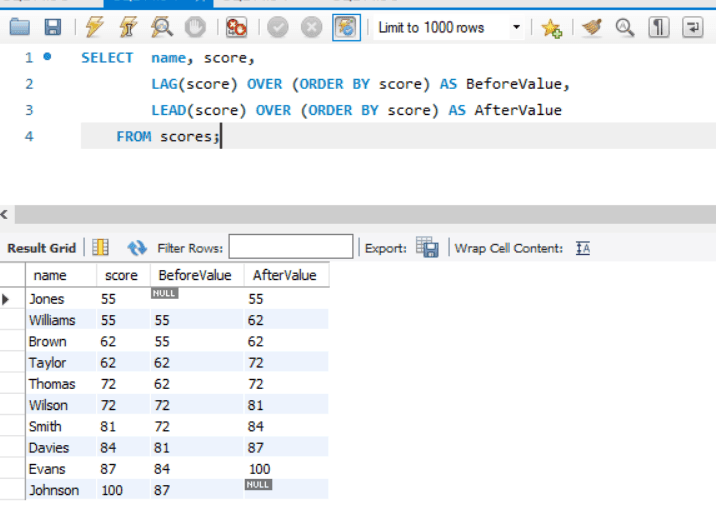##### FIRST_VALUE():
• The FIRST_VALUE() window function returns the value of the specified expression with respect to the first row in the window frame.
• Return value: any element [The data type of the return value matches that of the input value].
• FIRST_VALUE(expression) – expression could be a column, an actual expression, or a subquery that will be assessed against the value of the tables first row of a result set’s sorted partition.

##### LAST_VALUE():
• The LAST_VALUE window function returns the value of the specified expression with respect to the last row in the window frame.
• Return value: any element [The data type of the return value matches that of the input value].
##### Output of the FIRST_VALUE() and LAST_VALUE() functions: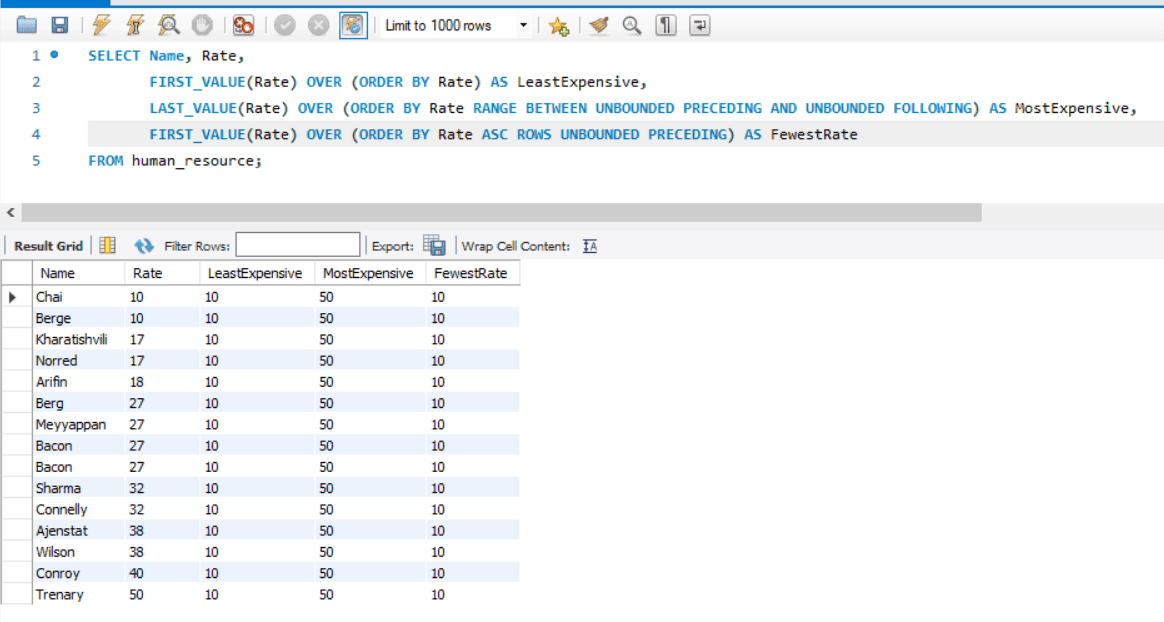##### NTILE():
• The NTILE() window function divides the rows for each window partition, as equally as possible, into a specified number of ranked groups which starting from 1.
• Return value: Int
• For example, if you wanted to mark the boundaries between the highest-ranking 20% of rows, the next-ranking 20% of rows, and so on, then you would use ntile(5).
• The NTILE(bucket) – The buckets represent the number of ranked teams. It will be a variety of associate expressions that evaluates to a positive number (greater than 0) for every partition. The buckets should not be nullable.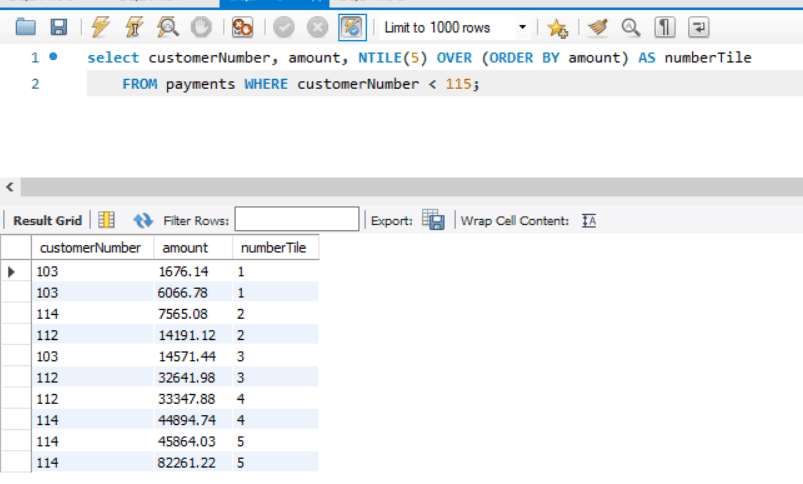##### RANK():
• The RANK window function determines the rank of a value in a group of values. Each value is ranked within its partition. Rows with equal values for the ranking criteria receive the same rank.
• Drill adds the number of tied rows to the tied rank to calculate the next rank and thus the ranks might not be consecutive numbers. For example, if two rows are ranked 1, the next rank is 3.

##### DENSE_RANK():
• The DENSE_RANK () window function determines the rank of a value in a group of values.
• Return value: bigint
• Each value is ranked within its partition. Rows with equal values receive the same rank.
• There are no gaps in the sequence of ranked values if two or more rows have the same rank.

##### ROW_NUMBER():
• The FIRST_VALUE() window function Return a unique integer for each row in a window, from a dense series that starts with 1, according to the emergent order that the window ORDER BY clause specifies.
• Return value: bigint
• For the two or more rows in a tie group, the unique values are assigned randomly.##### PERCENT_RANK():
• The PERCENT_RANK () window function calculates the percent rank of the current row. using the following formula:
• (rank – 1) / (number of rows in window partition – 1) where x is the rank of the current row
• Return value: double precision
• The lowest value of percent rank() within the window will always be 0.0 & highest possible value of percent_rank() within the window is 1.0.

Output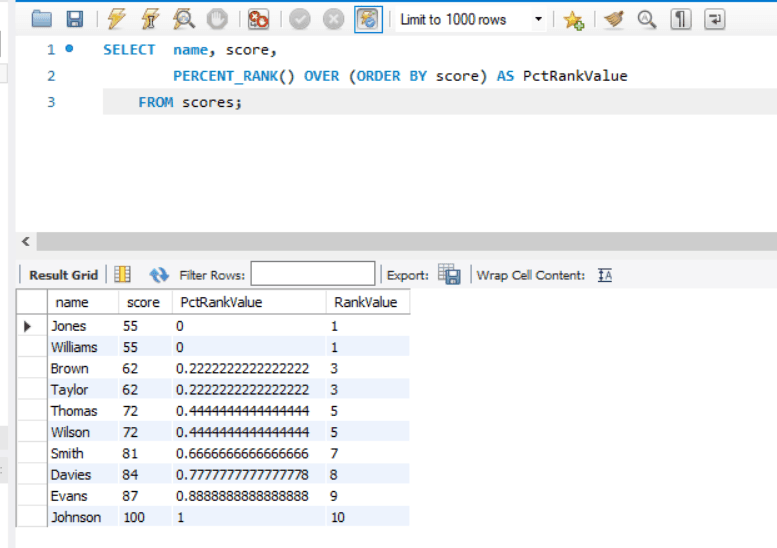##### CUME_DIST():
• The CUME_DIST() window function calculates the relative rank of the current row within a window partition:
• (number of rows preceding or peer with current row) / (total rows in the window partition).
• Return value: double precision
• Return a value that represents the number of rows with values less than or equal to the current row’s value divided by the total number of rows – in short, the relative position of the value in a set of values.

Output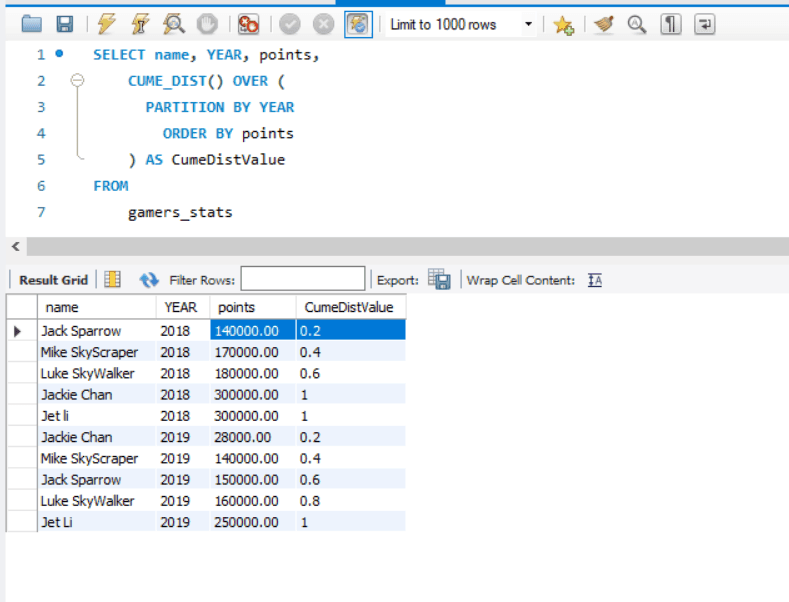For many analytical projects, MySQL can be an effective solution as it has the ideal database environment to implement analytical projects. A perfect fit for entry level startups and mid-sized firms to get started with data analytics. Moreover, it can also be scaled and optimized for larger applications.

Keep Learning!

Mr. Ashish Patel